# Print

7 Sep 201713 minutes to read

This feature is used to produce text and graphics from a computer screen or file into a sheet of paper. You can use `AllowPrinting` property in `PrintSettings` to enable/disable the print operation.

You have following options in printing.

• Print Sheet / Print Selected Area.
• Print with Gridlines

You can print the sheet or print the selected area by following ways,

The following code example describes the above behavior.

``````@Html.EJ().Button("btnPrint").Size(ButtonSize.Medium).ShowRoundedCorner(true).Width("80").ClientSideEvents(e => e.Click("printClick")).Text("Print")
@Html.EJ().Button("btnPrintSel").Size(ButtonSize.Medium).ShowRoundedCorner(true).ClientSideEvents(e => e.Click("printSelClick")).Text("Print Selected")

.PrintSettings(printOption => {
printOption.AllowPrinting(true);
})
.Sheets(sheet =>
{
sheet.RangeSettings(range =>
{
})
)

<script type="text/javascript">
function printClick(args) {
excelObj.XLPrint.printSheet();
}
function printSelClick(args) {
excelObj.XLPrint.printSelection();
}
</script>``````
``````namespace MVCSampleBrowser.Controllers
{
public partial class SpreadsheetController : Controller
{
public ActionResult Default()
{
List<ItemDetail> lItems = new List<ItemDetail>();
lItems.Add(new ItemDetail() { ItemName = "Casual Shoes", Date = new DateTime(2014, 02, 14), Time = new DateTime(2014, 02, 14, 11, 34, 32), Quantity = 10, Price = 20, Amount = 200, Discount = 1, Profit = 10 });
lItems.Add(new ItemDetail() { ItemName = "Sports Shoes", Date = new DateTime(2014, 06, 11), Time = new DateTime(2014, 06, 11, 05, 56, 32), Quantity = 20, Price = 30, Amount = 600, Discount = 5, Profit = 50 });
lItems.Add(new ItemDetail() { ItemName = "Formal Shoes", Date = new DateTime(2014, 07, 27), Time = new DateTime(2014, 07, 27, 03, 32, 44), Quantity = 20, Price = 15, Amount = 300, Discount = 7, Profit = 27 });
lItems.Add(new ItemDetail() { ItemName = "Sandals & Floaters", Date = new DateTime(2014, 11, 21), Time = new DateTime(2014, 11, 21, 06, 23, 54), Quantity = 15, Price = 20, Amount = 300, Discount = 11, Profit = 67 });
lItems.Add(new ItemDetail() { ItemName = "Flip- Flops & Slippers", Date = new DateTime(2014, 06, 23), Time = new DateTime(2014, 06, 23, 12, 43, 59), Quantity = 30, Price = 10, Amount = 300, Discount = 10, Profit = 70 });
ViewBag.Datasource = lItems;
return View();
}
}
}``````

The following output is displayed as a result of the above code example.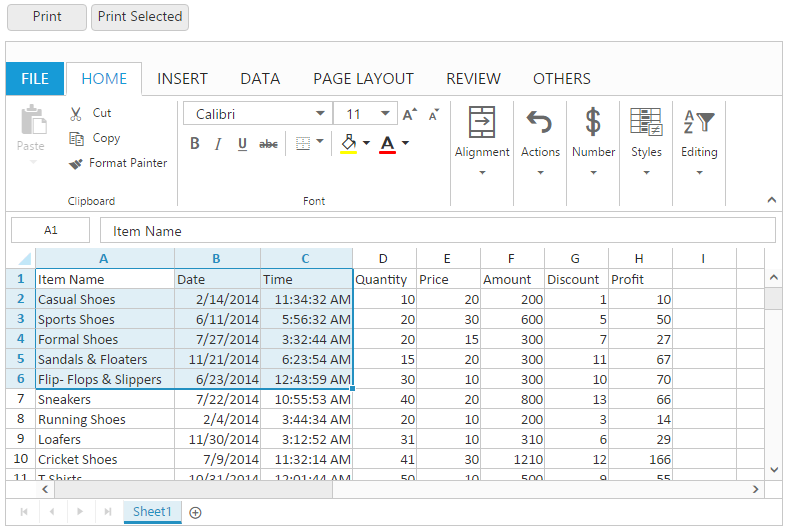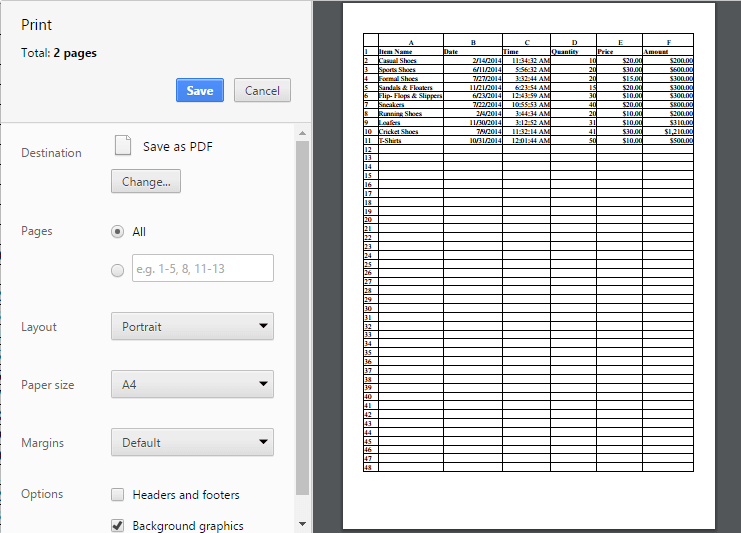Print dialog with entire sheet in Chrome browser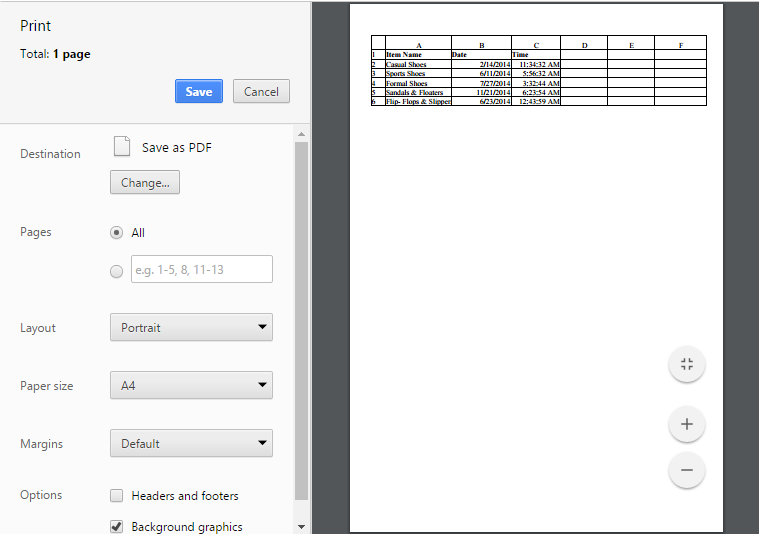Print dialog with selected area print in chrome browser

You have an option to print the spreadsheet with gridlines or without gridlines. You can do this by one of the following ways,

• Check or uncheck the Gridlines option under Show group of PAGE LAYOUT tab in ribbon to print with Gridlines.
• Using `ShowGridlines` property to enable / disable the gridlines.

The following code example describes the above behavior.

``````@(Html.EJ().Spreadsheet<object>("Spreadsheet")
.Sheets(sheet =>
{
sheet.RangeSettings(range =>
{
})
)``````
``````namespace MVCSampleBrowser.Controllers
{
public partial class SpreadsheetController : Controller
{
public ActionResult Default()
{
List<ItemDetail> lItems = new List<ItemDetail>();
lItems.Add(new ItemDetail() { ItemName = "Casual Shoes", Date = new DateTime(2014, 02, 14), Time = new DateTime(2014, 02, 14, 11, 34, 32), Quantity = 10, Price = 20, Amount = 200, Discount = 1, Profit = 10 });
lItems.Add(new ItemDetail() { ItemName = "Sports Shoes", Date = new DateTime(2014, 06, 11), Time = new DateTime(2014, 06, 11, 05, 56, 32), Quantity = 20, Price = 30, Amount = 600, Discount = 5, Profit = 50 });
lItems.Add(new ItemDetail() { ItemName = "Formal Shoes", Date = new DateTime(2014, 07, 27), Time = new DateTime(2014, 07, 27, 03, 32, 44), Quantity = 20, Price = 15, Amount = 300, Discount = 7, Profit = 27 });
lItems.Add(new ItemDetail() { ItemName = "Sandals & Floaters", Date = new DateTime(2014, 11, 21), Time = new DateTime(2014, 11, 21, 06, 23, 54), Quantity = 15, Price = 20, Amount = 300, Discount = 11, Profit = 67 });
lItems.Add(new ItemDetail() { ItemName = "Flip- Flops & Slippers", Date = new DateTime(2014, 06, 23), Time = new DateTime(2014, 06, 23, 12, 43, 59), Quantity = 30, Price = 10, Amount = 300, Discount = 10, Profit = 70 });
ViewBag.Datasource = lItems;
return View();
}
}
}``````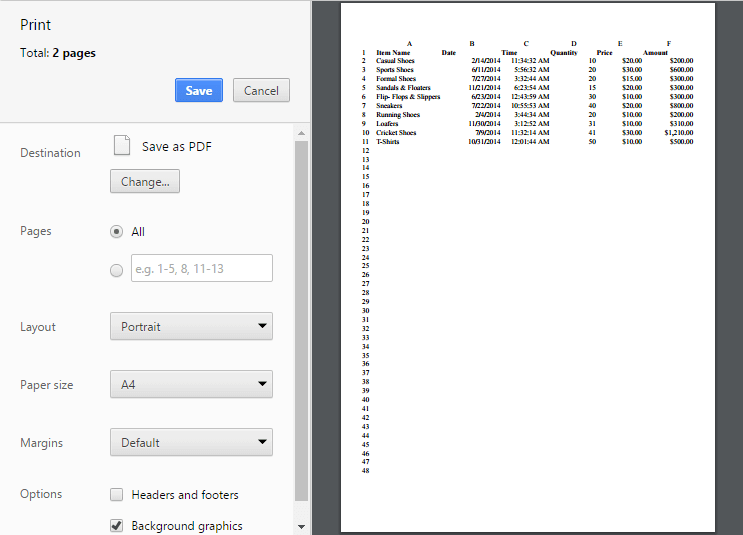Print dialog without gridlines in chrome browser

You have an option to print the spreadsheet with headers or without headers. You can do this by one of the following ways,

• Check or uncheck the Headings option under Show group of PAGE LAYOUT tab in ribbon.
• Using `ShowHeadings` property to enable / disable showHeadings.

The following code example describes the above behavior.

``````@(Html.EJ().Spreadsheet<object>("Spreadsheet")
.Sheets(sheet =>
{
sheet.RangeSettings(range =>
{
})
)``````
``````namespace MVCSampleBrowser.Controllers
{
public partial class SpreadsheetController : Controller
{
public ActionResult Default()
{
List<ItemDetail> lItems = new List<ItemDetail>();
lItems.Add(new ItemDetail() { ItemName = "Casual Shoes", Date = new DateTime(2014, 02, 14), Time = new DateTime(2014, 02, 14, 11, 34, 32), Quantity = 10, Price = 20, Amount = 200, Discount = 1, Profit = 10 });
lItems.Add(new ItemDetail() { ItemName = "Sports Shoes", Date = new DateTime(2014, 06, 11), Time = new DateTime(2014, 06, 11, 05, 56, 32), Quantity = 20, Price = 30, Amount = 600, Discount = 5, Profit = 50 });
lItems.Add(new ItemDetail() { ItemName = "Formal Shoes", Date = new DateTime(2014, 07, 27), Time = new DateTime(2014, 07, 27, 03, 32, 44), Quantity = 20, Price = 15, Amount = 300, Discount = 7, Profit = 27 });
lItems.Add(new ItemDetail() { ItemName = "Sandals & Floaters", Date = new DateTime(2014, 11, 21), Time = new DateTime(2014, 11, 21, 06, 23, 54), Quantity = 15, Price = 20, Amount = 300, Discount = 11, Profit = 67 });
lItems.Add(new ItemDetail() { ItemName = "Flip- Flops & Slippers", Date = new DateTime(2014, 06, 23), Time = new DateTime(2014, 06, 23, 12, 43, 59), Quantity = 30, Price = 10, Amount = 300, Discount = 10, Profit = 70 });
ViewBag.Datasource = lItems;
return View();
}
}
}``````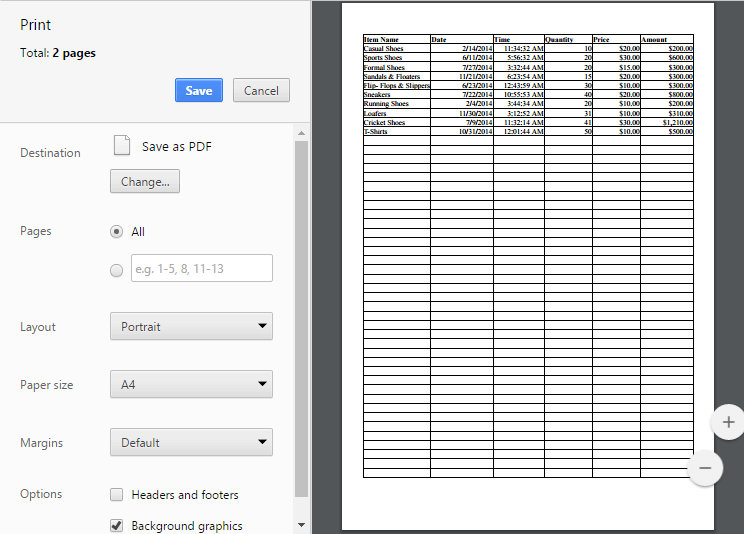Print dialog without headings in chrome browser